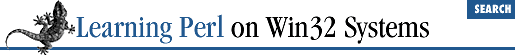home | O'Reilly's CD bookshelfs | FreeBSD | Linux | Cisco | Cisco Exam

### 4.5 The for Statement

Another Perl iteration construct is the ``` for``` statement, which looks suspiciously like C's or Java's ``` for``` statement, and works roughly the same way. Here it is:

```for ( ```

initial_exp
```
; ```

test_exp
```
; ```

re-init_exp
```
) {
```

statement_1
```
;
```

statement_2
```
;
```

statement_3
```
;
}```

Unraveled into forms we've seen before, this construct turns out as:

``````

initial_exp
```
;
while (```

test_exp
```
) {
```

statement_1
```
;
```

statement_2
```
;
```

statement_3
```
;
```

re-init_exp
```
;
}```

In either case, the ``` initial_exp ``` expression is evaluated first. This expression typically assigns an initial value to an iterator variable, but there are no restrictions on what it can contain; in fact, it may even be empty (doing nothing). Then the ``` test_exp ``` expression is evaluated for truth or falsehood. If the value is true, the body is executed, followed by the ``` re-init_exp ``` (typically, but not solely, used to increment the iterator). Perl then reevaluates the ``` test_exp ``` , repeating as necessary.

This example prints the numbers 1 through 10, each followed by a space:

```for (\$i = 1; \$i <= 10; \$i++) {
print "\$i ";
}```

Initially, the variable ``` \$i``` is set to 1. Then, this variable is compared with 10, which it is indeed less than or equal to. The body of the loop (the single ``` print``` statement) is executed, and then the re-init expression (the autoincrement expression ``` \$i++``` ) is executed, changing the value in ``` \$i``` to 2. Because this value is still less than or equal to 10, we repeat the process until the last iteration in which the value of 10 in ``` \$i``` gets changed to 11. The value is then no longer less than or equal to 10, so the loop exits (with ``` \$i``` having a value of 11).4.4 The do {} while/until Statement4.6 The foreach Statement